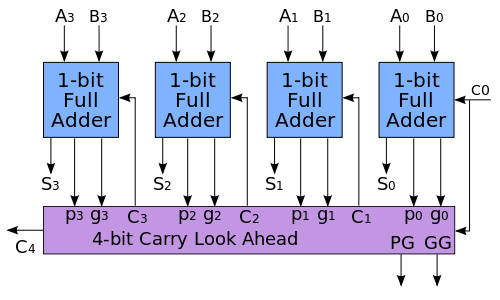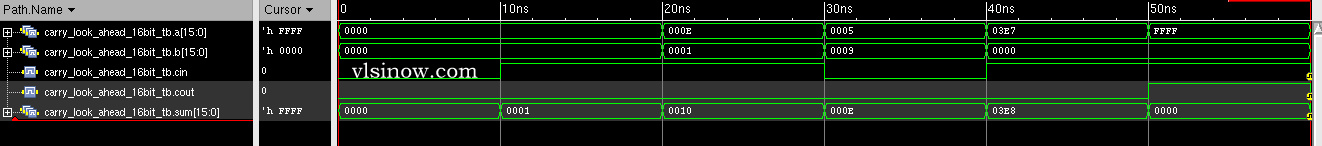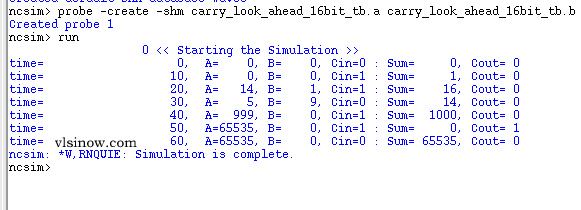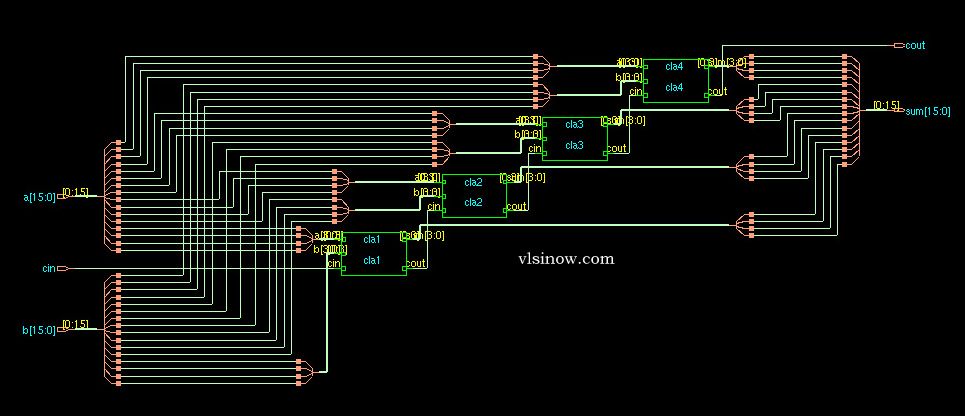The Verilog Code for 16-bit Carry Look Ahead Adder is given below-

```///////////////////////////////////////////////////////////
///////////////////////////////////////////////////////////

`timescale 1ns / 1ps
input [15:0] a,b;
input cin;
output [15:0] sum;
output cout;
wire c1,c2,c3;

carry_look_ahead_4bit cla1 (.a(a[3:0]), .b(b[3:0]), .cin(cin), .sum(sum[3:0]), .cout(c1));
carry_look_ahead_4bit cla2 (.a(a[7:4]), .b(b[7:4]), .cin(c1), .sum(sum[7:4]), .cout(c2));
carry_look_ahead_4bit cla3(.a(a[11:8]), .b(b[11:8]), .cin(c2), .sum(sum[11:8]), .cout(c3));
carry_look_ahead_4bit cla4(.a(a[15:12]), .b(b[15:12]), .cin(c3), .sum(sum[15:12]), .cout(cout));

endmodule

////////////////////////////////////////////////////////
////////////////////////////////////////////////////////

input [3:0] a,b;
input cin;
output [3:0] sum;
output cout;

wire [3:0] p,g,c;

assign p=a^b;//propagate
assign g=a&b; //generate

//carry=gi + Pi.ci

assign c=cin;
assign c= g|(p&c);
assign c= g | (p&g) | p&p&c;
assign c= g | (p&g) | p&p&g | p&p&p&c;
assign cout= g | (p&g) | p&p&g | p&p&p&g | p&p&p&p&c;
assign sum=p^c;

endmodule```

```////Testbench
reg [15:0] a,b;
reg cin;
wire [15:0] sum;
wire cout;

initial begin
a=0; b=0; cin=0;
#10 a=16'd0; b=16'd0; cin=1'd1;
#10 a=16'd14; b=16'd1; cin=1'd1;
#10 a=16'd5; b=16'd0; cin=1'd0;
#10 a=16'd999; b=16'd0; cin=1'd1;
end

initial
\$monitor( "A=%d, B=%d, Cin= %d, Sum=%d, Cout=%d", a,b,cin,sum,cout);
endmodule```Cadence RTL compiler is used to synthesize the verilog  code with Uofu standard library.  We got the following schematic after mapping the hdl code to the standard library. This is basically the synthesized view.If you have any query/suggestion please feel free to comment below the post.View all posts by admin →
Article Rating
Subscribe
Notify ofInline FeedbacksMiles
5 years ago

In 4-bit carry look ahead, one line is incorrect:

**incorrect** assign cout= g | (p&g) | p&p&g | p&p&p&p&c;
**Corrected** assign cout= g | (p&g) | p&p&g | p&p&p&g | p&p&p&p&c;

This was probably a typographical error, but I want to point it out for anyone having trouble using this code4 years ago

I did it. code is correctmonika Dnayneshwar kedar
3 years ago

I am trying this code in the Xilinx_ISE_DS_Win_14.7_1015_1 but iam not getting the ouput.Mathieu Martel
2 years ago

Code is wrong and testbench is very poor quality for testing no wonder there are bugs in design you need to fix your main 16bit adder. Person below linked edaplayground code, you can modify it and see it doesnt work. A=3635, B=77d4 testbench should get correct answer of 0ae09 but the dut returns 0ad09. Top-level 16bit should not send carry from 4bit to each other in c1c2c3c4, you need to reuse carry select unit logic to generate c0 c1 c2 c3 instead with p and g from 4-bit adders.rehmat
2 years ago